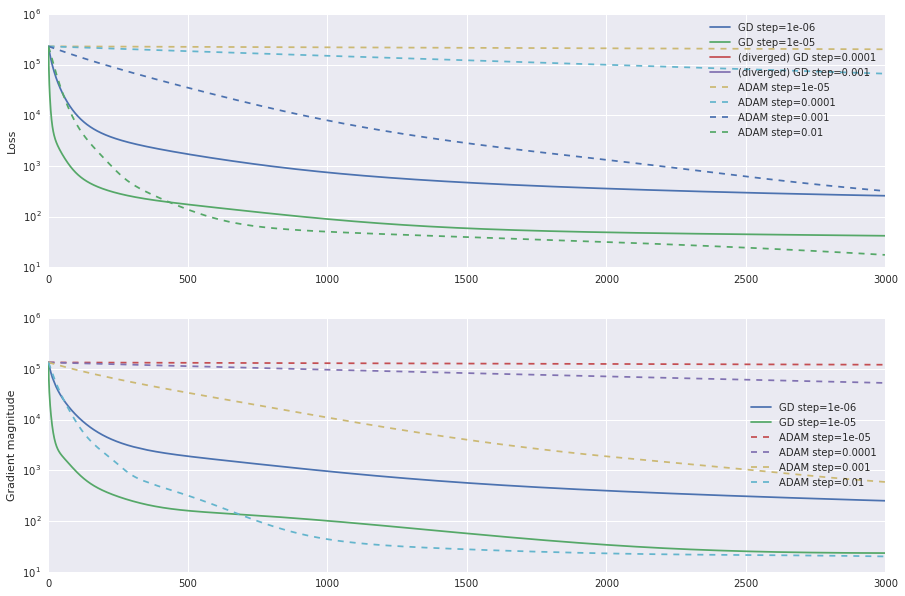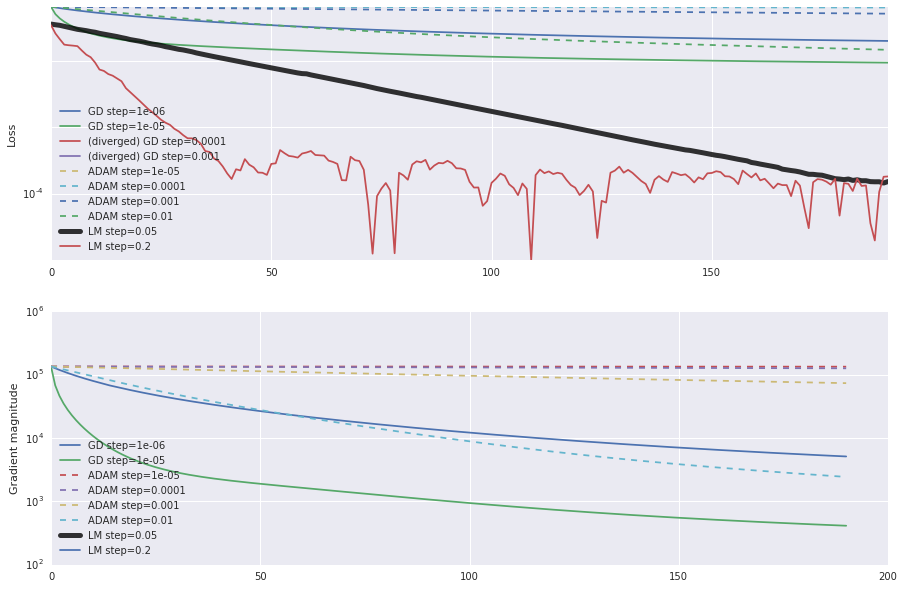# Gradient Descent Doesn't Find a Local Minimum in a Two Layer Linear Net¶

$$\newcommand{\E}{\mathop{\mathbb{E}}} \newcommand{\L}{\mathop{\mathcal{L}}}$$

I'll fit a two layer neural net on a realizable problem.

The loss function to find matrices $W_1$ and $W_2$ that minimize

\begin{align} \L(W_1, W_2) &= \E_{x \sim \mathcal{N}(0,\; I)} \|W_1 W_2 x - y\|^2 \\ y &= Ax \end{align}

where $A$ is a badly conditioned matrix ($\kappa = 10^5$).

This problem is matrix factorization in thin disguise: \begin{align} \L &= \E \|(W_1W_2 -A)x\|^2 \\ &= \text{tr}\; (W_1W_2-A)\left(\E xx^T\right)(W_1W_2-A)^T \\ &= \|W_1W_2-A\|_F^2. \end{align}

In :
import tensorflow as tf
import matplotlib.pylab as plt
import numpy as np
from scipy import sparse

In :
class TwoLayerLinearNet(object):

def __init__(self, name, X, Ytrue, W1_initial, W2_initial):
W1 = tf.Variable(W1_initial, trainable=True)
W2 = tf.Variable(W2_initial, trainable=True)

# Yhat = W1 W2 X
Yhat = tf.matmul(W1, tf.matmul(W2, X))
self.variables = (W1, W2)

# loss = || Yhat - Ytrue ||^2
loss = tf.reduce_sum(tf.squared_difference(Yhat, Ytrue))
self.loss = loss

# a tensor that stores the sum of the frobenius norms of the gradients of
# the loss wrt to the parameters
tf.reduce_sum(dLdvar**2)
]))

# this optimizer must be set before calling run_optimizer
self.optimizer_op = None
self.name = name
self.losses = []
self.plot_kwargs = {}

def run_optimizer(self, sess, num_steps):
self.losses = []

for it in xrange(num_steps):
self.loss,
self.optimizer_op
])

self.losses.append(loss)

# stop iterating if the loss has diverged
if not np.isfinite(self.losses[-1]):
break

def __init__(self, learning_rate, *args, **kwargs):
TwoLayerLinearNet.__init__(self, 'GD step=%g' % learning_rate,
*args, **kwargs)

learning_rate).minimize(self.loss, var_list=self.variables)

def __init__(self, learning_rate, *args, **kwargs):
*args, **kwargs)

learning_rate).minimize(self.loss, var_list=self.variables)
self.plot_kwargs = {'ls': '--'}

In :
def train_nets(xdim=6, wdim=6, ydim=10, nsamples=1000,
A_condition_number=1e-5):
W1_initial = np.random.randn(ydim, wdim)
W2_initial = np.random.randn(wdim, xdim)

# the true map from x to y
Atrue = np.linspace(1, A_condition_number, ydim
).reshape(-1, 1) * np.random.rand(ydim, xdim)
# the inputs
X = np.random.randn(xdim, nsamples)
# the y's to fit
Ytrue = Atrue.dot(X)

nets = [
for learning_rate in [1e-6, 1e-5, 1e-4, 1e-3]
] + [
for learning_rate in [1e-5, 1e-4, 1e-3, 1e-2]
]

with tf.Session() as sess:
sess.run(tf.global_variables_initializer())

for net in nets:
net.run_optimizer(sess, 3000)

return nets, (X, Ytrue, W1_initial, W2_initial)
nets, initial_params = train_nets()

In :
def plot_nets(nets, show_to_max=-1):
fig = plt.figure(figsize=(15, 10))

for net in nets:
show_to = -1 if show_to_max > len(net.losses) else show_to_max

if np.isfinite(net.losses[show_to]):
ax1.semilogy(net.losses[:show_to], label=net.name, **net.plot_kwargs)
**net.plot_kwargs)
else:
ax1.semilogy(net.losses[:1], marker='x', label='(diverged) ' + net.name,
**net.plot_kwargs)

ax1.set_ylabel('Loss')
ax1.legend(loc='best')
ax2.legend(loc='best')
return ax1, ax2

plot_nets(nets, -1);# Is This Really a Problem?¶

The optimization problem ought to reach $\L = 0$ (the problem is realizable). The loss seems to go down by a few orders of magnitude, but it does not reach 0 to machine precision.

It's tempting to say that the optimization has reached some kind of noise floor for the data set: perhaps adding more data points solves the issue. Not so. Minimizing the expected value of the loss, $\|W_1 W_2 - A\|$, produces similar curves. What we're orbserving has nothing to do with statistics of $\L$. It's entirely about the dynamics of gradient descent on product chains.

# What A Better Optimizer Could Do¶

Levenberg-Marquardt is the simplest optimizer I can think of that does not require second order information. We'll take steps $(\delta W_1, \delta W_2)$ that minimize an approximation to $\L$:

\begin{align} \L(W_1+\delta W_1, W_2+\delta W_2) &= \E_x \|(W_1+\delta W_1)(W_2+\delta W_2)x - y\|^2 \\ &\approx \E_x \|(\delta W_1\; W_2+W_1\;\delta W_2)x + \underbrace{W_1 W_2 x- y}_e\|^2 \\ &= \E_x \left\|\begin{bmatrix}(W_2x)^T \otimes I, & x^T\otimes W_1\end{bmatrix}\begin{bmatrix}\delta \vec{W}_1 \\ \delta \vec{W}_2\end{bmatrix} + e\right\|^2. \end{align}

We'll look for the minimizing $(\delta W_1, \delta W_2)$ by solving this least-squares problem. The algorithm looks a lot like K-FAC or Natural Gradient.

In :
def unpack_vector(x, *lengths):
"""Split up a sequence into several sequences of the given length.

Args:
x: a sequence
lengths: a sequence of n lengths.
Returns:
A list of sequences v1...vN. vi has length lengths[i].
"""
vectors = []
i = 0
for l in lengths:
v = x[i:i+l]
vectors.append(v)
i += l
assert i == len(x)
return vectors

class LevenbergMarquardt(object):

def __init__(self, learning_rate, X, Ytrue, W1_initial, W2_initial):
self.learning_rate = learning_rate
self.name = "LM step=%g" % learning_rate
self.X = X
self.Ytrue = Ytrue
self.W1 = W1_initial
self.W2 = W2_initial
self.plot_kwargs = {}

def take_step(self):
"Form and solve the least squares problem above about W1, W2."

Yhat = self.W1.dot(self.W2.dot(self.X))
RHS = -(Yhat - self.Ytrue).T.flatten()

LHS = np.hstack((
np.kron(self.W2.dot(self.X).T, np.eye(self.W1.shape)),
np.kron(self.X.T, self.W1)
))

# solve the least squares problem and parse the solution into matrices
dW1dW2 = np.linalg.lstsq(LHS, RHS)
dW1, dW2 = unpack_vector(dW1dW2, self.W1.size, self.W2.size)
dW1 = dW1.reshape(self.W1.T.shape).T
dW2 = dW2.reshape(self.W2.T.shape).T

# apply the update to the iterates
self.W1 = self.W1 + self.learning_rate * dW1
self.W2 = self.W2 + self.learning_rate * dW2

def loss(self):
Yhat = self.W1.dot(self.W2.dot(self.X))
return np.sum(Yhat - self.Ytrue)**2

def run_optimizer(self, num_steps):
self.losses = []
for _ in xrange(num_steps):
self.take_step()
self.losses.append(self.loss())

def train_lm(X, Ytrue, W1_initial, W2_initial):
nets = []
for step_sizes in [.05, .2]:
net = LevenbergMarquardt(step_sizes, X, Ytrue, W1_initial, W2_initial)
net.run_optimizer(200)
nets.append(net)
return nets

nets_lm = train_lm(*initial_params)

In :
nets_lm.plot_kwargs = {'lw': 5, 'color': 'k', 'alpha': .8}
ax1, _ = plot_nets(nets + nets_lm, 191);
ax1.set_yticks(10**np.linspace(-14, 6, 7))
ax1.axis('tight')

Out:
(0.0, 190.0, 4.5169799963375946e-08, 228241.57703817962)